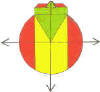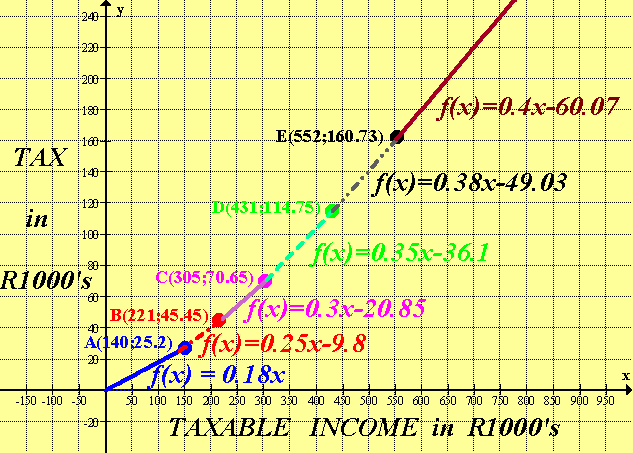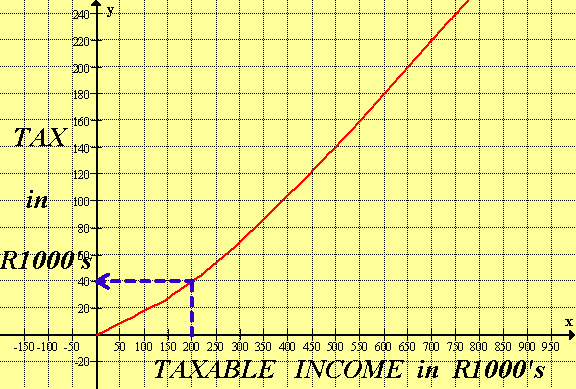DMD   EDU-HOME

WELCOME TO THIS PAGE INTENDED FOR STUDENTS AT THE UNIVERSITY OF THE WESTERN CAPE REGISTERED  FOR THE MODULE:

SARS 2010-2011 TAX TABLES

GRAPHICAL REPRESENTATIONNOTE:  R0 – R57 000  :  NO TAX PAYABLE. (UNDER 65 years)

since TAXABLE INCOME = R57 000

SARS Income Tax Tables

1 March 2010 - 28 February 2011

 Taxable Income Tax Rate R 0 - R 140 000 18% R 140 001 - R 221 000 R 25 200 + (25% of amount above R 140 000) R 221 001 - R 305 000 R 45 450 + (30% of amount above R 221 000) R 305 001 - R 431 000 R 70 650 + (35% of amount above R 305 000) R 431 001 - R 552 000 R 114 750 + (38% of amount above R 431 000) R 552 001 and above R 160 730 + (40% of amount above R 552 000)
 Primary Rebate R 10 260 Additional Rebate (Persons 65 and older) R 5 675

CALCULATION USING OFF EQUATIONS (ROUGH ESTIMATE ONLY)

EXAMPLE 1:

TAXABLE AMOUNT = R200 000

TAX PAYABLE  =  R40 200, which is calculated thus:

0.25 multiplied by 200 ( NB: which is in Thousands -  9.8

= 50 – 9.8 = 40.2 ( in Thousands)

READING TAX OFF GRAPH (ROUGH ESTIMATE ONLY)

EXAMPLE 2

TAXABLE AMOUNT = R200 000

TAX PAYABLE = R40 000 (approximately)EXAMPLE 3

Use the given equations in the tax graphs to verify the points of intersection A, B, C, D and E.

For  A (140 ; 25.2)Now calculate B, C, D and E similarly.

©  DESMOND DESAI, DMD EDU-HOME, 2010

All rights reserved. Designed and created by Desmond Desai, South Africa. This page is protected by Copyright. No part of this page may be reproduced, stored in a retrieval system, or transmitted in any form of by any means, electronic, mechanical, photocopying, recording or otherwise, without the prior written permission of the copyright holder.